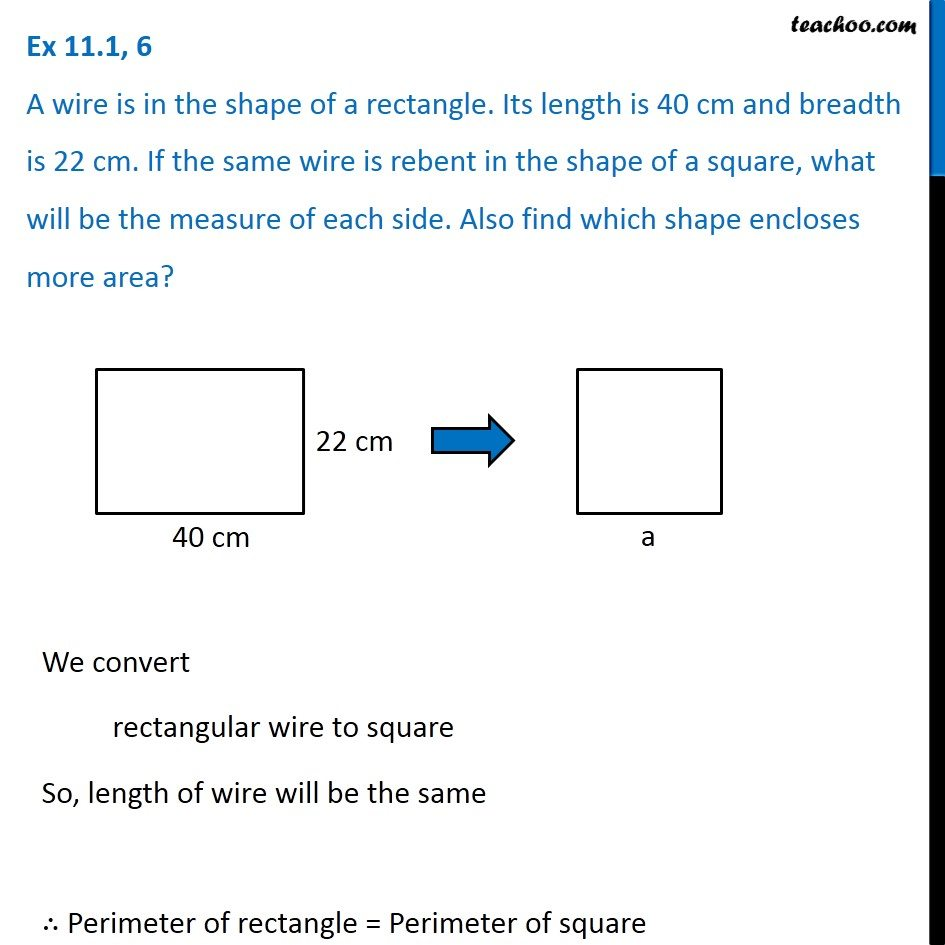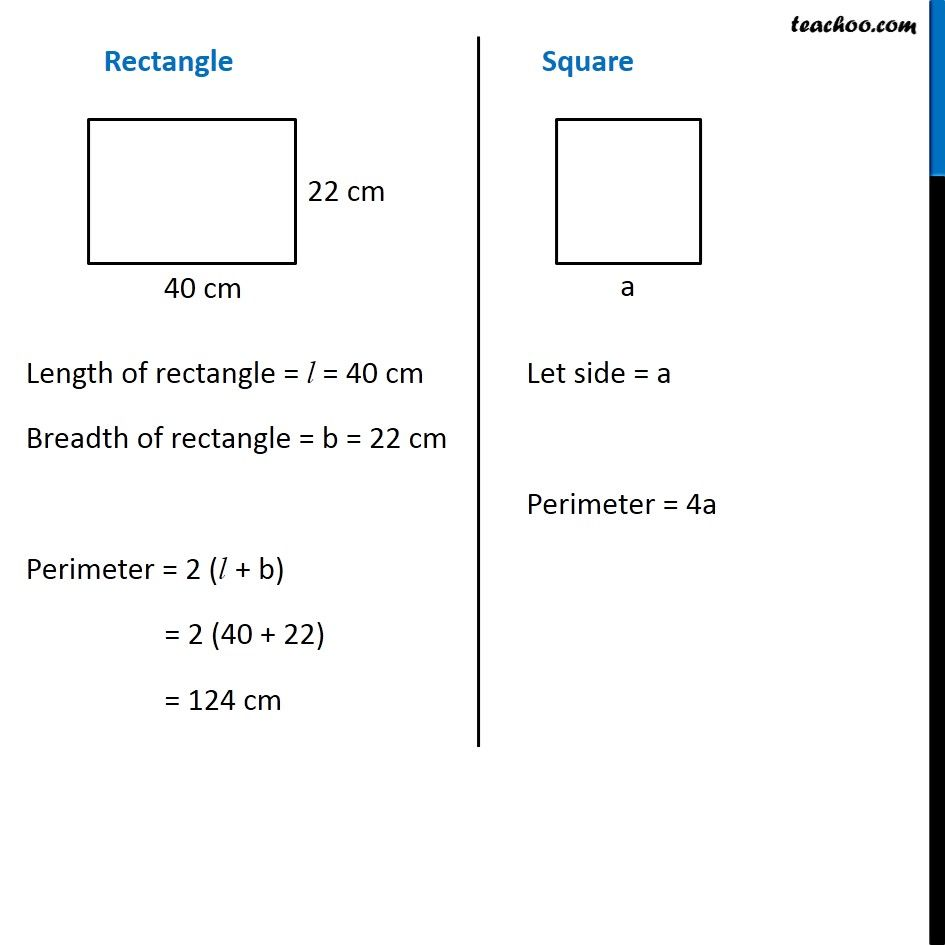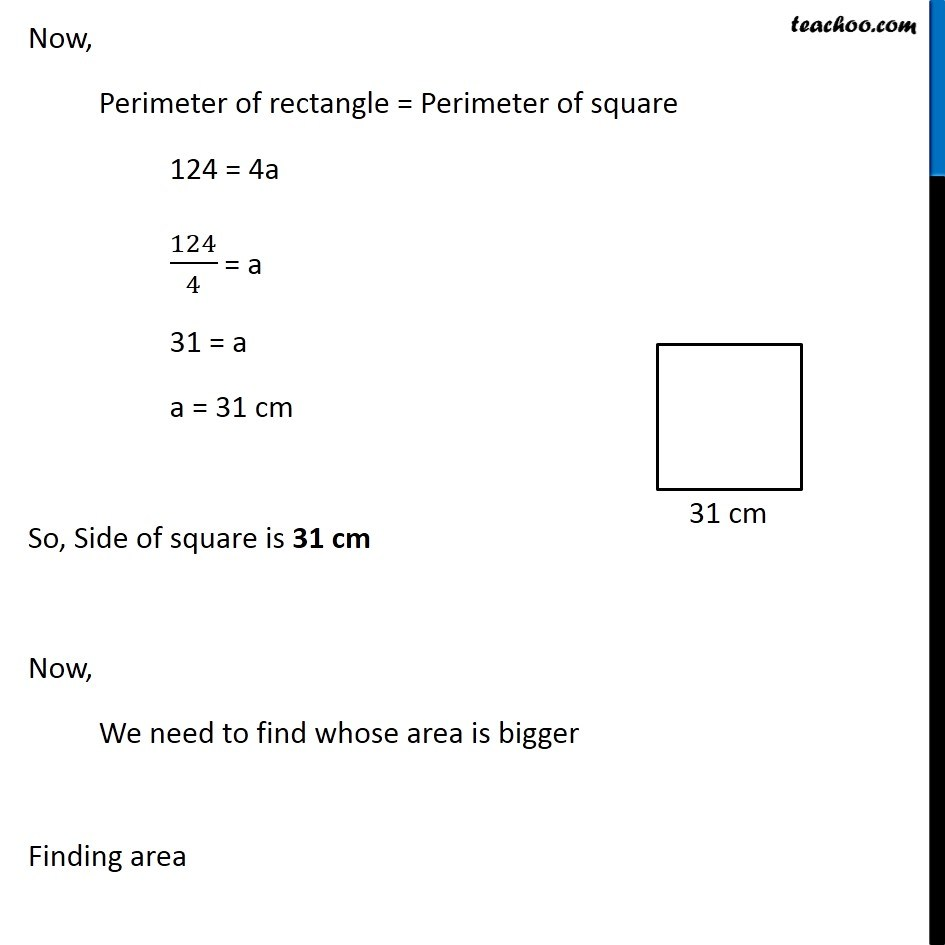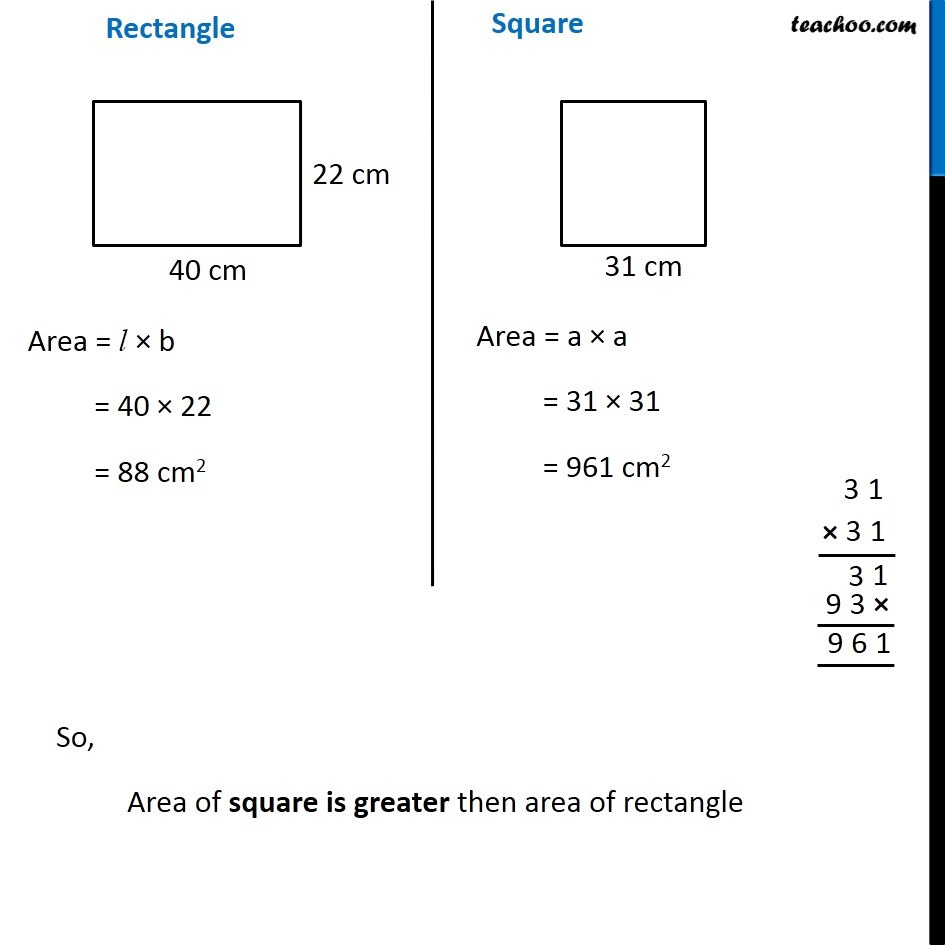1. Chapter 11 Class 7 Perimeter and Area
2. Concept wise
3. Converting Square to Rectangle

Transcript

Ex 11.1, 6 A wire is in the shape of a rectangle. Its length is 40 cm and breadth is 22 cm. If the same wire is rebent in the shape of a square, what will be the measure of each side. Also find which shape encloses more area? We convert rectangular wire to square So, length of wire will be the same ∴ Perimeter of rectangle = Perimeter of square Rectangle Length of rectangle = l = 40 cm Breadth of rectangle = b = 22 cm Perimeter = 2 (l + b) = 2 (40 + 22) = 124 cm Square Let side = a Perimeter = 4a Now, Perimeter of rectangle = Perimeter of square 124 = 4a 124/4 = a 31 = a a = 31 cm So, Side of square is 31 cm Now, We need to find whose area is bigger Finding area Rectangle Area = l × b = 40 × 22 = 88 cm2 Square Area = a × a = 31 × 31 = 961 cm2 So, Area of square is greater then area of rectangle